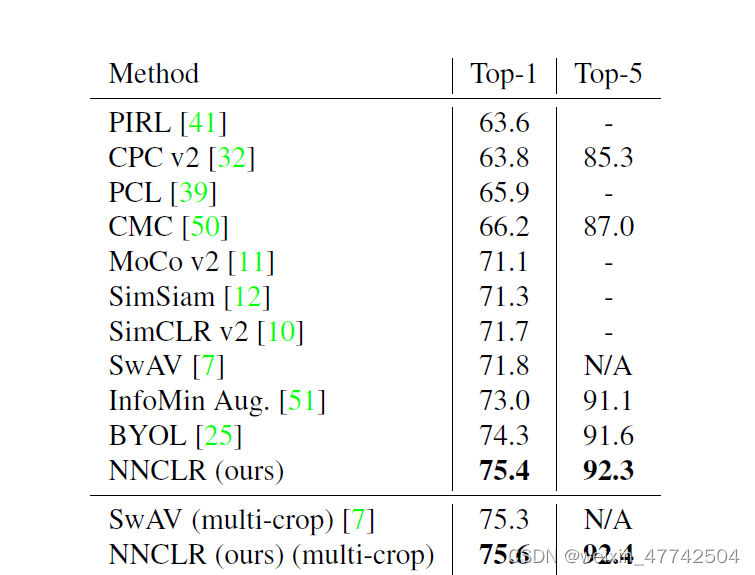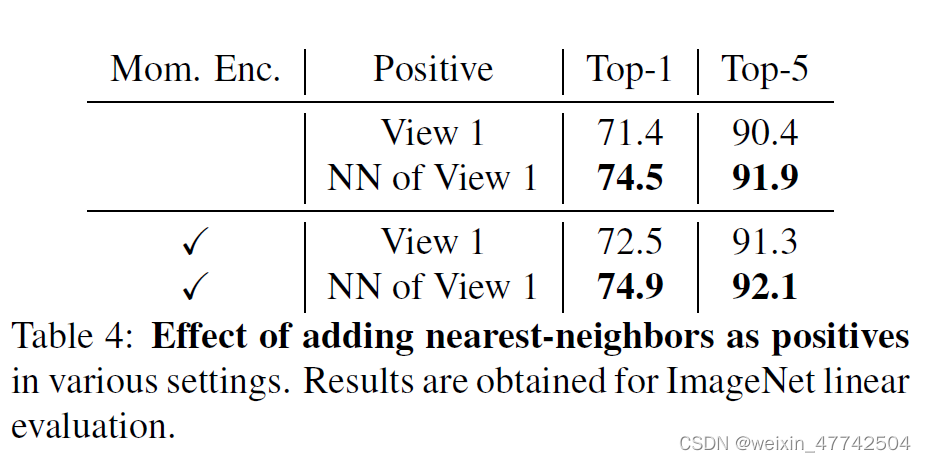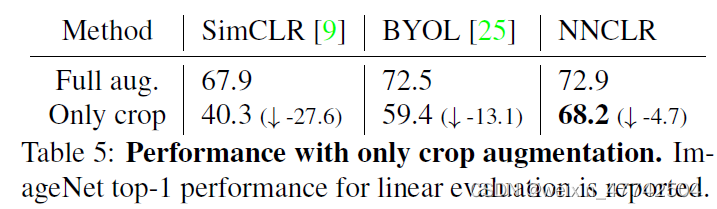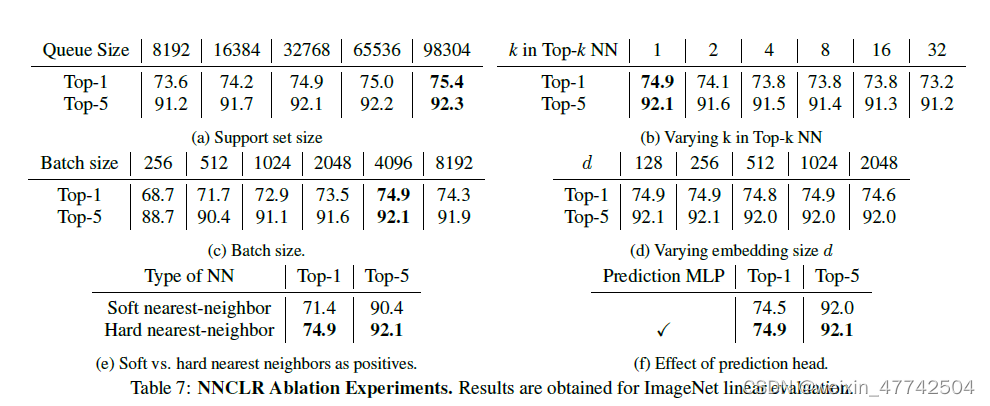# With a Little Help from My Friends: Nearest-Neighbor Contrastive Learning of Visual Representations¶

### 2. 背景介绍¶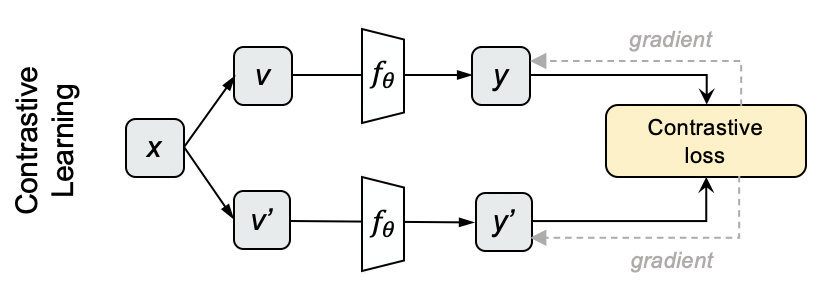• 采样N个图片，用不同的数据增强方法为每个图片生成两个view

• 分别将他们输入网络，获得编码表示y和y’。

• 对上下两批表示两两计算cosine，得到NxN的矩阵，每一行的对角线位置代表y和y’的相似度，其余代表y和N-1个负例的相似度。对每一行做softmax分类，采用交叉熵损失作为loss，就得到对比学习的损失了。

MoCo采用的对比学习损失函数就是InfoNCE loss，以此来训练模型，公式如下：

\mathrm{L}_{\mathrm{y}}=-\log \frac{\exp \left(\mathrm{y} \cdot \mathrm{y}^{\prime} / \tau\right)}{\sum_{\mathrm{i}=0}^{\mathrm{N}} \exp \left(\mathrm{y} \cdot \mathrm{y}^{\prime} / \tau\right)}

$\tau$ 表示温度系数。

• 数据扩充的组合在有效的预测任务中起着关键作用；
• 在表征和对比损失之间引入可学习的非线性变换，大大提高了学习表示的质量；
• 与有监督的学习相比，对比学习需要更大batch size和训练轮数。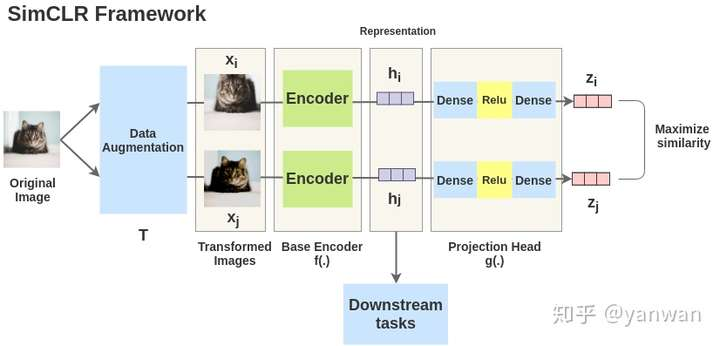loss function为：

\mathcal{L}_{i}^{\operatorname{SimCLR}}=-\log \frac{\exp \left(z_{i} \cdot z_{i}^{+} / \tau\right)}{\sum_{k=1}^{n} \exp \left(z_{i} \cdot z_{k}^{+} / \tau\right)}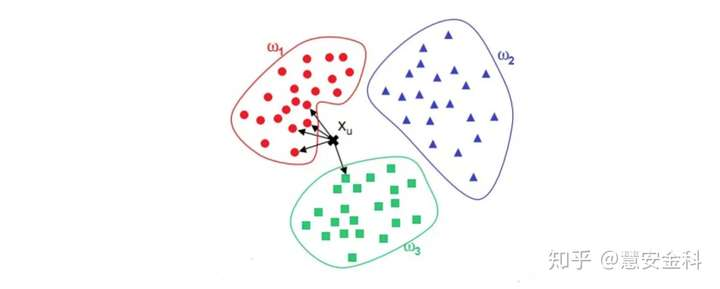KNN（K-Nearest Neighbor）算法是机器学习算法中最基础、最简单的算法之一。它既能用于分类，也能用于回归。KNN通过测量不同特征值之间的距离来进行分类。对于任意n维输入向量，分别对应于特征空间中的一个点，输出为该特征向量所对应的类别标签或预测值。

KNN应该是最简单最经典的无监督算法，而对比学习（Contrastive Learning）则是当下比较流行的无监督学习范式。本文将这两者结合，取得了非常有意思的效果。

https://zhuanlan.zhihu.com/p/158023072

https://zhuanlan.zhihu.com/p/197802321

https://zhuanlan.zhihu.com/p/45453761

### 3. 介绍¶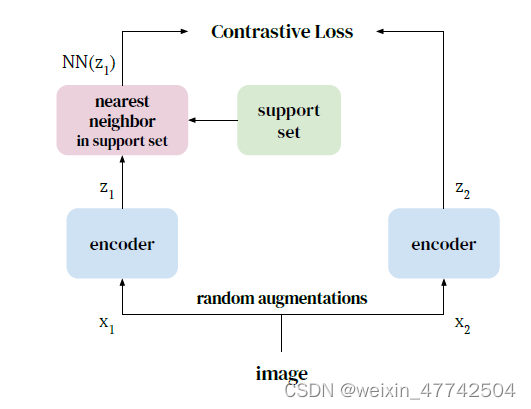### 4. 方法¶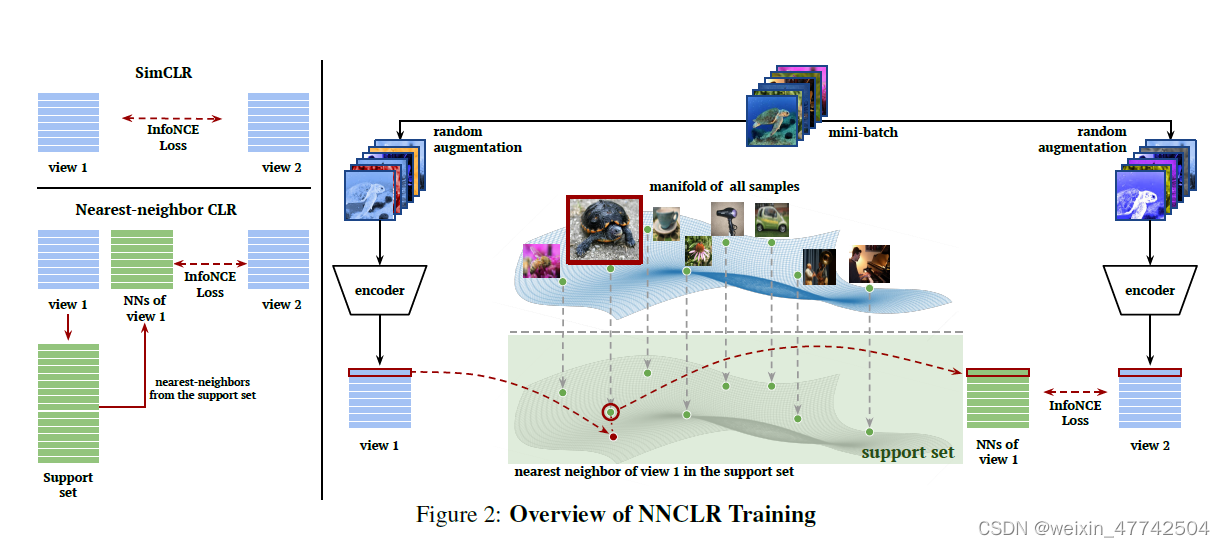\mathcal{L}_{i}^{\mathrm{NNCLR}}=-\log \frac{\exp \left(\mathrm{NN}\left(z_{i}, Q\right) \cdot z_{i}^{+} / \tau\right)}{\sum_{k=1}^{n} \exp \left(\mathrm{NN}\left(z_{i}, Q\right) \cdot z_{k}^{+} / \tau\right)}

NN表示选取最近邻的操作符：

\mathrm{NN}(z, Q)=\underset{q \in Q}{\arg \min }\|z-q\|_{2}

### 5. 实验分析¶